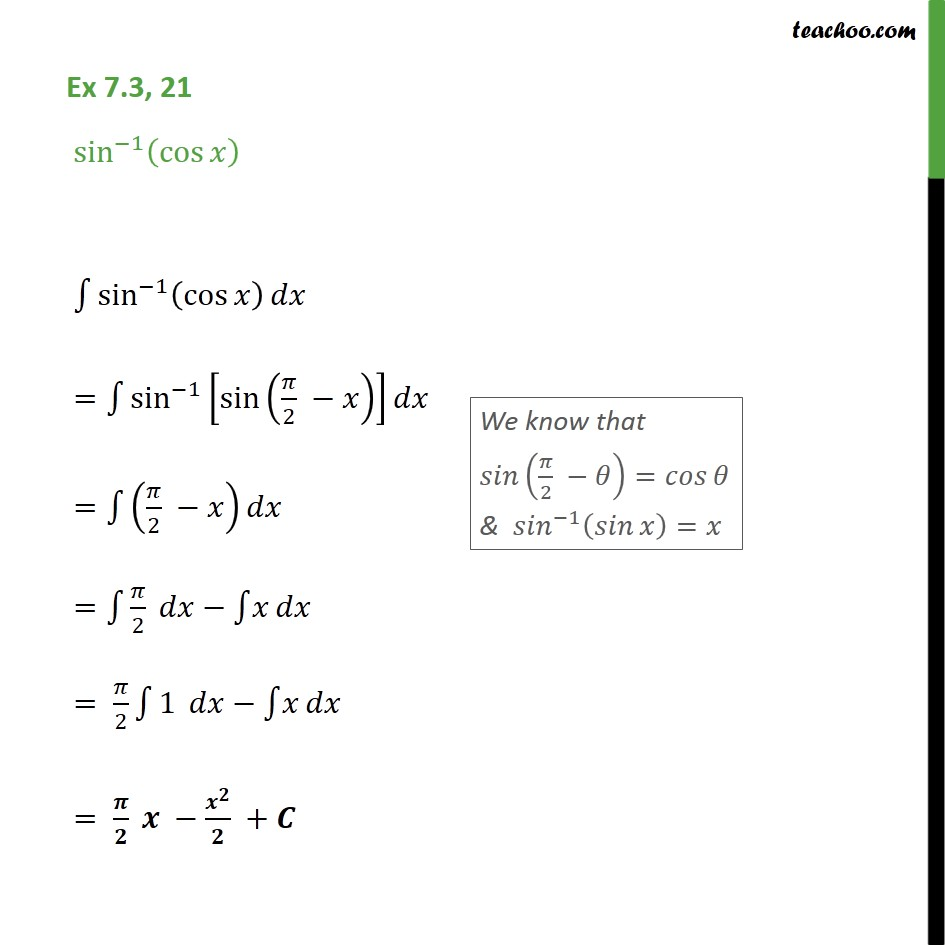1. Chapter 7 Class 12 Integrals (Term 2)
2. Concept wise
3. Integration using trigo identities - Inv Trigo formulae

Transcript

Ex 7.3, 21 sin 1 cos sin 1 cos = sin 1 sin 2 = 2 = 2 = 2 1 = +

Integration using trigo identities - Inv Trigo formulae